# HSPT Math : Concepts

## Example Questions

### Example Question #6 : Decimals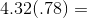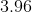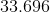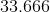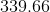Explanation:

Multiply normally and remember that the decimal point is four places from the right.

### Example Question #7 : Decimals

Express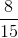as a decimal.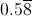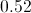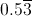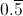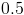Explanation:

Divide 8 by 15: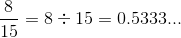The 3 repeats, so the correct choice is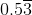.

### Example Question #8 : Decimals

Give the decimal equivalent of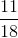.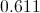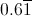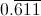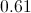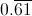Explanation:

Divide 11 by 18: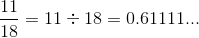The 1 repeats infinitely, so this can be rewritten as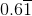.

### Example Question #9 : Decimals

Give the decimal equivalent of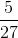.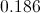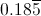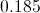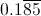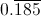Explanation:

Divide 5 by 27: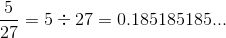The group "185" repeats infinitely, so this can be written as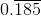.

### Example Question #10 : Decimals

Give the decimal equivalent of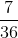.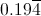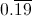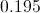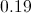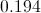Explanation:

Divide 7 by 36: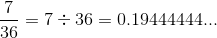The 4 repeats infinitely, so this can be rewritten as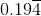.

### Example Question #1 : How To Find Percentage

A watch originally cost $55.00. If it is on sale for 20% off, what is the sale price of the watch? Possible Answers: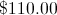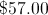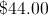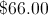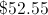Correct answer:Explanation: To solve: First, find what is 20% of 55 by multiplying: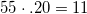Then, subtract 20% (11) from the original price: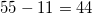The final sale price will be$44.00.

### Example Question #2 : How To Find Percentage

Which is largest?

a. 30% of 150

b. 20% of 300

c. 40% of 60

d. 10% of 400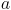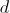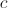Explanation:

To solve:

First find the corresponding percentage of each multiple choice answer,

a)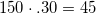b)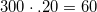c)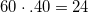d)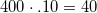Then, compare the amounts and select which amount is the largest:

b) 60

### Example Question #3 : How To Find Percentage

How do you writeas a percent?

50%

20%

5%

15%

25%

20%

Explanation:is equal to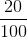, which is 20%.

### Example Question #4 : How To Find Percentage

Andrew has completed 80% of the race. If the race is 5000 meters, how far along is Andrew?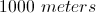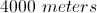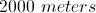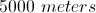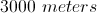Explanation:

Multiply the length of the race by the percentage accomplished by Andrew: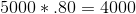Answer: Andrew has completed 4000 meters of the race.

### Example Question #5 : How To Find Percentage

Which is less?

a. 60% of 10

b. 20% of 40

c. 30% of 20

d. 10% of 50Explanation:

Find the amounts by multiplying each number to the percentage rate: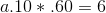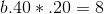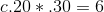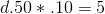Compare the amounts to find the least. The answer is D.

### All HSPT Math Resources